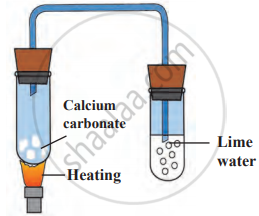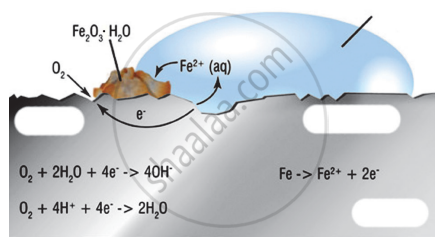# SCERT Maharashtra Question Bank solutions for 10th Standard SSC Science and Technology 1 Maharashtra State Board 2021 chapter 3 - Chemical reactions and equations [Latest edition]

#### Chapters## Chapter 3: Chemical reactions and equations

Choose the correct option.Answer in one sentence.Right or Wrong sentenceWrite an ExplanationWrite scientific reasonsSolve the following QuestionsExplain the following reactions with the balanced equationsAnswer the FollowingAnswer the following questions
Choose the correct option.

### SCERT Maharashtra Question Bank solutions for 10th Standard SSC Science and Technology 1 Maharashtra State Board 2021 Chapter 3 Chemical reactions and equations Choose the correct option.

#### Choose the correct option.

Choose the correct option. | Q 1

Writing a chemical reaction in brief by using chemical formulae is called as _______.

• chemical change

• chemical symbol

• chemical equation

• chemical reaction

Choose the correct option. | Q 2

When the positive charge on an ion increases or the negative charge on them decreases it is called as _______.

• reduction

• corrosion

• oxidation

• decomposition

Choose the correct option. | Q 3

The chemical reaction in which two or more products are formed from a single reactant is called _______ reaction.

• decomposition

• combination

• displacement

• double displacement

Choose the correct option. | Q 4

In the chemical equation the _______ are written on the left-hand side.

• products

• reactants

• element

• catalyst

Choose the correct option. | Q 5

Choose the correct option from the bracket and explain the statement giving a reason.
(Oxidation, displacement, electrolysis, reduction, zinc, copper, double displacement, decomposition)

Addition of an aqueous solution of ZnSO4 to an aqueous solution of BaCl2 is an example of ______ reaction.

### SCERT Maharashtra Question Bank solutions for 10th Standard SSC Science and Technology 1 Maharashtra State Board 2021 Chapter 3 Chemical reactions and equations Answer in one sentence.

#### Answer the following question in one sentence.

Answer in one sentence. | Q 1

Write chemical formula for rust.

Answer in one sentence. | Q 2

Complete the given chemical reaction.

$\ce{CuSO_{4(aq)} + Fe_{(s)} ->}$______ + ______

Right or Wrong sentence

### SCERT Maharashtra Question Bank solutions for 10th Standard SSC Science and Technology 1 Maharashtra State Board 2021 Chapter 3 Chemical reactions and equations Right or Wrong sentence

Right or Wrong sentence | Q 1

If edible oil is left aside for a long time, it will get rancid.

• Right

• Wrong

Write an Explanation

### SCERT Maharashtra Question Bank solutions for 10th Standard SSC Science and Technology 1 Maharashtra State Board 2021 Chapter 3 Chemical reactions and equations Write an Explanation

Write an Explanation | Q 1

Write an Explanation.

Balanced equations

Write an Explanation | Q 2

Write an Explanation.

Endothermic reaction

Write scientific reasons

### SCERT Maharashtra Question Bank solutions for 10th Standard SSC Science and Technology 1 Maharashtra State Board 2021 Chapter 3 Chemical reactions and equations Write scientific reasons

Write scientific reasons | Q 1

Write scientific reason.

Always paint grills of doors and windows before using them.

Write scientific reasons | Q 2

Give scientific reason.

It is reccommended to use air tight container for storing oil for long time.

Write scientific reasons | Q 3

Give scientific reason.

When the gas formed on heating limstone is passed through freshly prepared lime water, the lime water turns milky.

Write scientific reasons | Q 4

Give scientific reason.

It takes time for pieces of Shahabad tile to disappear in HCl, but its powder disappears rapidly.

Write scientific reasons | Q 5

Write scientific reason.

Adding zinc particles to a solution of copper sulphate makes the blue solution colorless.

Solve the following Questions

### SCERT Maharashtra Question Bank solutions for 10th Standard SSC Science and Technology 1 Maharashtra State Board 2021 Chapter 3 Chemical reactions and equations Solve the following Questions

Solve the following Questions | Q 1

Explain the factors affecting the rate of chemical reaction with example.

Solve the following Questions | Q 2.1

What are reactants? Explain with the help of example.

Solve the following Questions | Q 2.2

What are products? Explain with the help of example.

Solve the following Questions | Q 3.1

Explain the following reaction with their balanced chemical equation.

Ammonia gas reacts with hydrogen chloride.

Solve the following Questions | Q 3.2

Explain the following reaction with their balanced chemical equation.

Hydrogen sulphide reacts with sulphur dioxide.

Solve the following Questions | Q 4.1

Classify the following reaction into Exothermic and Endothermic reactions.

Magnesium ribbon when burned in air, it forms magnesium oxide.

• Exothermic reaction

• Endothermic reaction

Solve the following Questions | Q 4.2

Identify the endothermic and exothermic reaction.

HCl + NaOH → NaCl + H2O + heat

• Exothermic reaction

• Endothermic reaction

Solve the following Questions | Q 4.3

Identify the endothermic and exothermic reaction.

$\ce{2KClO_{3(s)}->[\Delta] 2KCl_{(s)} + 3O2}$

• Exothermic reaction

• Endothermic reaction

Solve the following Questions | Q 4.4

Identify the endothermic and exothermic reaction.

$\ce{CaO + H2O -> Ca(OH)2 + Heat}$

• Exothermic reaction

• Endothermic reaction

Solve the following Questions | Q 4.5

Identify the endothermic and exothermic reaction.

$\ce{CaCO_{3(s)}->[\Delta] CaO_{(s)} + CO2}$

• Exothermic reaction

• Endothermic reaction

Explain the following reactions with the balanced equations

### SCERT Maharashtra Question Bank solutions for 10th Standard SSC Science and Technology 1 Maharashtra State Board 2021 Chapter 3 Chemical reactions and equations Explain the following reactions with the balanced equations

Explain the following reactions with the balanced equations | Q 1

Explain the following reaction with the balanced equation.

Aluminium reacts with dil hydrochloric acid

Explain the following reactions with the balanced equations | Q 2

Explain the following reaction with the balanced equation.

Reaction of zinc with dil hydrochloric acid

### SCERT Maharashtra Question Bank solutions for 10th Standard SSC Science and Technology 1 Maharashtra State Board 2021 Chapter 3 Chemical reactions and equations Answer the Following

Answer the Following | Q 1

Match the columns.

 Reactants Products Types of chemical reaction 1. MgH2 → Mg + H2 Endothermic 2. 2H2S + SO2 → 3S + 2H2O Oxidation 3. CaO + H2O → Ca(OH)2 + heat Exothermic Redox
Answer the Following | Q 2

Write three steps of writing chemical equations with example?

Answer the Following | Q 3.1

Identify from the following reaction the reactants that undergo oxidation and reduction.

2Ag2O → 4 Ag + O2 ↑

Answer the Following | Q 3.2

Identify from the reaction the reactants that undergo oxidation and reduction.

2Mg + O2 → 2MgO

Answer the Following | Q 3.3

Identify from the reaction the reactants that undergo oxidation and reduction.

$\ce{NiO + H2 -> Ni + H2O}$

Answer the Following | Q 4.1

What is corrosion?

Answer the Following | Q 4.2

What is electrolysis?

Answer the Following | Q 4.3

Which changes occur during chemical changes?

Answer the Following | Q 4.4

What is the reaction called when oxidation and reduction take place simultaneously? Explain with one example.

Answer the Following | Q 5

Explain the reaction given in the figure.### SCERT Maharashtra Question Bank solutions for 10th Standard SSC Science and Technology 1 Maharashtra State Board 2021 Chapter 3 Chemical reactions and equations Answer the following questions

Answer the following questions | Q 1.1

Balance the given chemical reaction as per the instructions below.

$\ce{NaOH + H2SO4 -> Na2SO4 + H2O}$

Write names of reactants and products of chemical reaction.

Answer the following questions | Q 1.2

Balance the given chemical reaction as per the instructions below.

$\ce{NaOH + H2SO4 -> Na2SO4 + H2O}$

Make a list of the elements in the chemical equation.

Answer the following questions | Q 1.3

Balance the given chemical reaction as per the instructions below.

$\ce{NaOH + H2SO4 -> Na2SO4 + H2O}$

Write the number of atoms of various elements in the reactants and products.

Answer the following questions | Q 1.4

Balance the given chemical reaction as per the instructions below.

$\ce{NaOH + H2SO4 -> Na2SO4 + H2O}$

Balance the equation with proper coefficient and rewrite the equation.

Answer the following questions | Q 1.5

Balance the given chemical reaction as per the instructions below.

$\ce{NaOH + H2SO4 -> Na2SO4 + H2O}$

Oxidation means losing electrons then what is reduction?

Answer the following questions | Q 2

Observe the following figure and write the answer of the question.1. Which process is shown in the figure?
2. Explain the chemical reaction shown in the figure.
3. Write the reactions on anode and cathode.

## Chapter 3: Chemical reactions and equations

Choose the correct option.Answer in one sentence.Right or Wrong sentenceWrite an ExplanationWrite scientific reasonsSolve the following QuestionsExplain the following reactions with the balanced equationsAnswer the FollowingAnswer the following questions## SCERT Maharashtra Question Bank solutions for 10th Standard SSC Science and Technology 1 Maharashtra State Board 2021 chapter 3 - Chemical reactions and equations

SCERT Maharashtra Question Bank solutions for 10th Standard SSC Science and Technology 1 Maharashtra State Board 2021 chapter 3 (Chemical reactions and equations) include all questions with solution and detail explanation. This will clear students doubts about any question and improve application skills while preparing for board exams. The detailed, step-by-step solutions will help you understand the concepts better and clear your confusions, if any. Shaalaa.com has the Maharashtra State Board 10th Standard SSC Science and Technology 1 Maharashtra State Board 2021 solutions in a manner that help students grasp basic concepts better and faster.

Further, we at Shaalaa.com provide such solutions so that students can prepare for written exams. SCERT Maharashtra Question Bank textbook solutions can be a core help for self-study and acts as a perfect self-help guidance for students.

Concepts covered in 10th Standard SSC Science and Technology 1 Maharashtra State Board 2021 chapter 3 Chemical reactions and equations are Classification of Change: Chemical Changes, Chemical Equation, Types of Chemical Change or Chemical Reaction, Direct Combination (or Synthesis) Reaction, Decomposition Reactions, Single Displacement Reactions, Double Displacement Reaction, Energy Change in Chemical Reactions, Rate of Chemical Reaction, Oxidation, Reduction and Redox Reactions, Corrosion of Metals and Its Prevention, Rancidity of Food and Its Prevention, Chemical Reaction, Balancing Chemical Equation, Factors Affecting the Rate of a Chemical Reaction.

Using SCERT Maharashtra Question Bank 10th Standard Board Exam solutions Chemical reactions and equations exercise by students are an easy way to prepare for the exams, as they involve solutions arranged chapter-wise also page wise. The questions involved in SCERT Maharashtra Question Bank Solutions are important questions that can be asked in the final exam. Maximum students of Maharashtra State Board 10th Standard Board Exam prefer SCERT Maharashtra Question Bank Textbook Solutions to score more in exam.

Get the free view of chapter 3 Chemical reactions and equations 10th Standard Board Exam extra questions for 10th Standard SSC Science and Technology 1 Maharashtra State Board 2021 and can use Shaalaa.com to keep it handy for your exam preparation